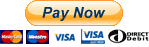Nauseating Pumpkin Mush

# npm

Learn about our RFC process, Open RFC meetings & more.Join in the discussion! »

## js-big-decimal

1.3.1 • Public • Published

# JS Big Decimal

Work with large numbers on the client side with high precision.

## Installation

### Usage

❗️ Note: Usage has changed since version 1.1.4

Require in javascript as

For typescript, use

For web, when used with script tag, a variable on Window object is created.

## Operations

### bigDecimal(number)

Create a new object of type BigDecimal. Supports parameters of type number and string. If string passed cannot be parsed as a number error is thrown. It is recommended to use string as it circumvents the issue of precision with JS native float implementation and max limit for integer.

It supports exponentiation, but only with integral exponent.

### getValue()

Returns the string value of the decimal.

### getPrettyValue(number, digits, separator)

By default this returns the number in standard number format, comma after every three digits. Both arguments, digits - the number of digits (of the integral part) to group by, and separator - the character to mark the separation. Example of this can be to format a 16 digit number as credit card.

Alternately, use the instance property. It returns the result as string.

### round(number, precision, roundingMode)

Returns the rounded value to the specified precision (number of digits after decimal). The default precision is set to 0 and rounding mode set to HALF_EVEN if no argument is passed.

Alternately, use the instance property. It returns the result as bigDecimal.

Passing in a negative argument for digits to round off to returns the nearest multiple of power of 10. If the magnitude of the argument is larger than or equal to the number of digits in the integral part of the number to round, zero is returned.

Round also supports the following rounding modes (These are same as that of Java 8):

• CEILING - Rounding mode to round towards positive infinity.
• DOWN - Rounding mode to round towards zero.
• FLOOR - Rounding mode to round towards negative infinity.
• HALF_DOWN - Rounding mode to round towards "nearest neighbor" unless both neighbors are equidistant, in which case round down.
• HALF_EVEN - Rounding mode to round towards the "nearest neighbor" unless both neighbors are equidistant, in which case, round towards the even neighbor.
• HALF_UP - Rounding mode to round towards "nearest neighbor" unless both neighbors are equidistant, in which case round up.
• UNNECESSARY (!Not Implemented!)- Rounding mode to assert that the requested operation has an exact result, hence no rounding is necessary.
• UP - Rounding mode to round away from zero.

Extensive description of the modes can be found at Rounding Modes

### floor(number)

Returns the whole number nearest but not greater than the input number.

The instance function returns the result as a new bigDecimal

### ceil(number)

Returns the whole number nearest but not lesser than the input number.

The instance function returns the result as a new bigDecimal

### compareTo(number1, number2)

Compare two numbers. Returns 1, 0 and -1 if number1 > number2, number1 == number2 and number1 < number2 respectively.

Alternately, use the instance property. It returns the result as Integer.

### negate(number)

Returns negation of a given number.

Alternately, use the instance property. It returns the result as new bigDecimal.

Add two numbers. Pass in negative for subtraction. Ensure parameters are strings.

Alternately, use the instance property. It returns the result as new bigDecimal.

### subtract(minuend, subtrahend)

Subtract one number from another

Alternately, use the instance property. It returns the result as new bigDecimal.

### multiply(multiplicand, multiplier)

Multiply two numbers. Ensure parameters are strings.

Alternately, use the instance property. It returns the result as new bigDecimal.

### divide(dividend, divisor, precision)

Divide two numbers. Pass arguments as string if calling on bigDecimal or pass an instance of bigDecimal if calling on object. precision is an optional parameter with default value of 8.

Alternately, use the instance property. It returns the result as new bigDecimal.

### modulus(dividend, divisor)

Get the modulus of two numbers, i.e., remainder when the dividend is divided by the divisor. Note that both divisor and dividend need to be integers.

Alternately, use the instance property. It returns the result as new bigDecimal.

Further, the result takes the sign of the dividend and the sign of the divisor is ignored. Note that this behaviour is the same as in Java and JavaScript.

# Support the developers ❤️ ⭐️ 💸

If this library helps you in your organization, you can show some love by giving the repo a star or support by making a nominal monetary contribution.$1.99$ 2.99$4.49$ 9.99## Keywords

### Install

npm i js-big-decimal

2,804

1.3.1

MIT

106 kB

12